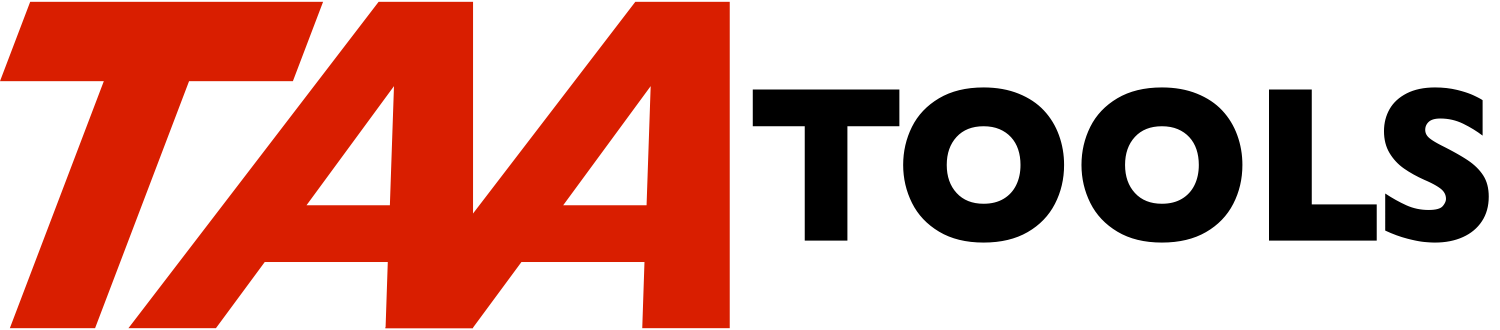`CLCMOD10        CALCULATE MODULUS 10                   TAACLQZ`
```
The Calculate  Modulus 10 command  calculates the  value for a  Modulus
10 Self-Check  Digit.  The  command returns a value  for the self-check
digit  which is  intended to be  added to a  number such  as a customer
number.  DDS supports the  CHECK(M10) keyword for a field to  assist in
ensuring the number is keyed correctly.

Self-check  digits  are commonly  used  when  entering  values such  as
customer   numbers   to  minimize   errors  caused   by   miskeying  or
transposing digits.

A different  algorithm is  required for  a UCC  modulus  10 self  check
digit.  See the CLCUCCCHKD tool.

The  code for  the CLCMOD10  command  calculates the  self check  digit
number  by  calculating from  the  units position.    This  follows the
algorithm used by the  system in the CHECK(M10)  function in DDS.   The
CLCMOD10L command  calculates beginning from  the high  order position.
Both  commands produce  the same  answer on  an  odd number  of digits.
The result differs when there are an even number of digits.

The  CLCMOD10 command  creates the self-check  digit.   For example, if
your customer  number is  5 digits  without the  self-check digit,  the
following  CL program  using  CLCMOD10 would  calculate the  self-check
digit and return a 6 digit customer number.

PGM        PARM(&CUST5 &CUST6)
DCL        &CUST5 *CHAR LEN(5)
DCL        &CUST6 *CHAR LEN(6)
DCL        &DIGIT *CHAR LEN(1)
CLCMOD10   INPUT(&CUST5) MOD10DGT(&DIGIT)
CHGVAR     &CUST6 (&CUST5 *CAT &DIGIT)
ENDPGM

The 6 digit value becomes the customer number.

See  the DDS  manual for a  discussion of  how the  self-check digit is
calculated.

Checking the value without using DDS
------------------------------------

You can use CLCMOD10 to  check a value also.   Just strip off and  save
the digit  position, use  CLCMOD10 to  calculate the self-check  digit,
and them compare the calculated digit to the saved version.

CLCMOD10  algorithm
-------------------

There is  a weight factor of 2 for beginning  at the units position and
working left every other digit.  Each  digit of the result is added  to
the sum.   Then  beginning at  the units  position -1, the  sum of  the

For example, the number 61248 would result in:

2 x 8  = 16       Digits = 1 and 6 = 7
2 x 2  = 4        Digits = 4       = 4
2 X 6  = 12       Digits = 1 and 2 = 3
--
14

Digits not multiplied   4 and 1    = 5
--
19

Next higher number ending in 0      20
Subtract (20 -19) = 1 as the self check digit

Using CLCMOD10L,  the self check digit would be  the same because there
are an odd number of digits.

If  there is an even  number of digits, such  as 123456, the result for
CLCMOD10 would be 6.

6 x 2 = 12      Digits   1 + 2 = 3
4 x 2 =  8      Digits   0 + 8 = 8
2 x 2 =  4      Digits   0 + 4 = 4
--
15
Digits     1 + 3 + 5     9
--
24

Next higher number ending in 0      30
Subtract (30 -24) = 6

Using CLCMOD10L, the result would be 9

1 x 2 =  2      Digits   0 + 2 = 2
3 x 2 =  6      Digits   0 + 6 = 6
5 x 2 = 10      Digits   1 + 0 = 1
--
9
Digits     2 + 4 + 6    12
--
21

Next higher number ending in 0      30
Subtract (30 -21) = 9

CLCMOD10  escape messages you can monitor for
---------------------------------------------

TAA9891    The input value cannot be all blanks.
TAA9892    The input value must only contain digits.

Escape messages from based on functions will be re-sent.

CLCMOD10L escape messages you can monitor for
---------------------------------------------

TAA9891    The input value cannot be all blanks.
TAA9892    The input value must only contain digits.

Escape messages from based on functions will be re-sent.

CLCMOD10 Command parameters                           *CMD
---------------------------

INPUT         The input  value without  the self-check  digit.   The
value cannot  be all blanks and must  have only digits
and  trailing   blanks.    Up  to  31  digits  may  be
entered.

MOD10DGT      The self-check  digit to  be returned.   The  variable
must be declared as *CHAR LEN(1).

CLCMOD10L Command parameters                          *CMD
----------------------------

INPUT         The input  value without  the self-check  digit.   The
value cannot  be all blanks and  must have only digits
and  trailing  blanks.    Up  to  31  digits  may   be
entered.

MOD10DGT      The self-check  digit to  be returned.   The  variable
must be declared as *CHAR LEN(1).

Restrictions
------------

Because the  commands return variables, they  may only be used  in a CL
program.

Prerequisites
-------------

The following TAA Tools must be on your system:

EDTVAR          Edit variable
SNDESCMSG       Send escape message

Implementation
--------------

None, the tool is ready to use.

Objects used by the tool
------------------------

Object        Type    Attribute      Src member    Src file
------        ----    ---------      ----------    ----------

CLCMOD10      *CMD                   TAACLQZ       QATTCMD
CLCMOD10L     *CMD                   TAACLQZ2      QATTCMD
TAACLQZC      *PGM       CLP         TAACLQZC      QATTCL
TAACLQZC      *PGM       CLP         TAACLQZC2     QATTCL
```

Added to TAA Productivity tools January 15, 2002